# Yes we Do!###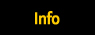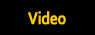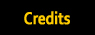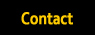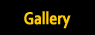<!-- /*==================================================* \$Id: slideshow.js,v 1.16 2003/10/14 12:39:00 pat Exp \$ Copyright 2000-2003 Patrick Fitzgerald http://slideshow.barelyfitz.com/ This program is free software; you can redistribute it and/or modify it under the terms of the GNU General Public License as published by the Free Software Foundation; either version 2 of the License, or (at your option) any later version. This program is distributed in the hope that it will be useful, but WITHOUT ANY WARRANTY; without even the implied warranty of MERCHANTABILITY or FITNESS FOR A PARTICULAR PURPOSE. See the GNU General Public License for more details. You should have received a copy of the GNU General Public License along with this program; if not, write to the Free Software Foundation, Inc., 59 Temple Place, Suite 330, Boston, MA 02111-1307 USA *==================================================*/ // There are two objects defined in this file: // "slide" - contains all the information for a single slide // "slideshow" - consists of multiple slide objects and runs the slideshow //================================================== // slide object //================================================== function slide(src,link,text,target,attr) { // This is the constructor function for the slide object. // It is called automatically when you create a new slide object. // For example: // s = new slide(); // Image URL this.src = src; // Link URL this.link = link; // Text to display this.text = text; // Name of the target window ("_blank") this.target = target; // Custom duration for the slide, in milliseconds. // This is an optional parameter. // this.timeout = 3000 // Attributes for the target window: // width=n,height=n,resizable=yes or no,scrollbars=yes or no, // toolbar=yes or no,location=yes or no,directories=yes or no, // status=yes or no,menubar=yes or no,copyhistory=yes or no // Example: "width=200,height=300" this.attr = attr; // Create an image object for the slide if (document.images) { this.image = new Image(); } // Flag to tell when load() has already been called this.loaded = false; //-------------------------------------------------- this.load = function() { // This method loads the image for the slide if (!document.images) { return; } if (!this.loaded) { this.image.src = this.src; this.loaded = true; } } //-------------------------------------------------- this.hotlink = function() { // This method jumps to the slide's link. // If a window was specified for the slide, then it opens a new window. var mywindow; // If this slide does not have a link, do nothing if (!this.link) return; // Open the link in a separate window? if (this.target) { // If window attributes are specified, // use them to open the new window if (this.attr) { mywindow = window.open(this.link, this.target, this.attr); } else { // If window attributes are not specified, do not use them // (this will copy the attributes from the originating window) mywindow = window.open(this.link, this.target); } // Pop the window to the front if (mywindow && mywindow.focus) mywindow.focus(); } else { // Open the link in the current window location.href = this.link; } } } //================================================== // slideshow object //================================================== function slideshow( slideshowname ) { // This is the constructor function for the slideshow object. // It is called automatically when you create a new object. // For example: // ss = new slideshow("ss"); // Name of this object // (required if you want your slideshow to auto-play) // For example, "SLIDES1" this.name = slideshowname; // When we reach the last slide, should we loop around to start the // slideshow again? this.repeat = true; // Number of images to pre-fetch. // -1 = preload all images. // 0 = load each image is it is used. // n = pre-fetch n images ahead of the current image. // I recommend preloading all images unless you have large // images, or a large amount of images. this.prefetch = -1; // IMAGE element on your HTML page. // For example, document.images.SLIDES1IMG this.image; // ID of a DIV element on your HTML page that will contain the text. // For example, "slides2text" // Note: after you set this variable, you should call // the update() method to update the slideshow display. this.textid; // TEXTAREA element on your HTML page. // For example, document.SLIDES1FORM.SLIDES1TEXT // This is a depracated method for displaying the text, // but you might want to supply it for older browsers. this.textarea; // Milliseconds to pause between slides. // Individual slides can override this. this.timeout = 3000; // Hook functions to be called before and after updating the slide // this.pre_update_hook = function() { } // this.post_update_hook = function() { } // These are private variables this.slides = new Array(); this.current = 0; this.timeoutid = 0; //-------------------------------------------------- // Public methods //-------------------------------------------------- this.add_slide = function(slide) { // Add a slide to the slideshow. // For example: // SLIDES1.add_slide(new slide("s1.jpg", "link.html")) var i = this.slides.length; // Prefetch the slide image if necessary if (this.prefetch == -1) { slide.load(); } this.slides[i] = slide; } //-------------------------------------------------- this.play = function(timeout) { // This method implements the automatically running slideshow. // If you specify the "timeout" argument, then a new default // timeout will be set for the slideshow. // Make sure we're not already playing this.pause(); // If the timeout argument was specified (optional) // then make it the new default if (timeout) { this.timeout = timeout; } // If the current slide has a custom timeout, use it; // otherwise use the default timeout if (typeof this.slides[ this.current ].timeout != 'undefined') { timeout = this.slides[ this.current ].timeout; } else { timeout = this.timeout; } // After the timeout, call this.loop() this.timeoutid = setTimeout( this.name + ".loop()", timeout); } //-------------------------------------------------- this.pause = function() { // This method stops the slideshow if it is automatically running. if (this.timeoutid != 0) { clearTimeout(this.timeoutid); this.timeoutid = 0; } } //-------------------------------------------------- this.update = function() { // This method updates the slideshow image on the page // Make sure the slideshow has been initialized correctly if (! this.valid_image()) { return; } // Call the pre-update hook function if one was specified if (typeof this.pre_update_hook == 'function') { this.pre_update_hook(); } // Convenience variable for the current slide var slide = this.slides[ this.current ]; // Determine if the browser supports filters var dofilter = false; if (this.image && typeof this.image.filters != 'undefined' && typeof this.image.filters != 'undefined') { dofilter = true; } // Load the slide image if necessary slide.load(); // Apply the filters for the image transition if (dofilter) { // If the user has specified a custom filter for this slide, // then set it now if (slide.filter && this.image.style && this.image.style.filter) { this.image.style.filter = slide.filter; } this.image.filters.Apply(); } // Update the image. this.image.src = slide.image.src; // Play the image transition filters if (dofilter) { this.image.filters.Play(); } // Update the text this.display_text(); // Call the post-update hook function if one was specified if (typeof this.post_update_hook == 'function') { this.post_update_hook(); } // Do we need to pre-fetch images? if (this.prefetch > 0) { var next, prev, count; // Pre-fetch the next slide image(s) next = this.current; prev = this.current; count = 0; do { // Get the next and previous slide number // Loop past the ends of the slideshow if necessary if (++next >= this.slides.length) next = 0; if (--prev < 0) prev = this.slides.length - 1; // Preload the slide image this.slides[next].load(); this.slides[prev].load(); // Keep going until we have fetched // the designated number of slides } while (++count < this.prefetch); } } //-------------------------------------------------- this.goto_slide = function(n) { // This method jumpts to the slide number you specify. // If you use slide number -1, then it jumps to the last slide. // You can use this to make links that go to a specific slide, // or to go to the beginning or end of the slideshow. // Examples: // onClick="myslides.goto_slide(0)" // onClick="myslides.goto_slide(-1)" // onClick="myslides.goto_slide(5)" if (n == -1) { n = this.slides.length - 1; } if (n < this.slides.length && n >= 0) { this.current = n; } this.update(); } //-------------------------------------------------- this.goto_random_slide = function(include_current) { // Picks a random slide (other than the current slide) and // displays it. // If the include_current parameter is true, // then // See also: shuffle() var i; // Make sure there is more than one slide if (this.slides.length > 1) { // Generate a random slide number, // but make sure it is not the current slide do { i = Math.floor(Math.random()*this.slides.length); } while (i == this.current); // Display the slide this.goto_slide(i); } } //-------------------------------------------------- this.next = function() { // This method advances to the next slide. // Increment the image number if (this.current < this.slides.length - 1) { this.current++; } else if (this.repeat) { this.current = 0; } this.update(); } //-------------------------------------------------- this.previous = function() { // This method goes to the previous slide. // Decrement the image number if (this.current > 0) { this.current--; } else if (this.repeat) { this.current = this.slides.length - 1; } this.update(); } //-------------------------------------------------- this.shuffle = function() { // This method randomly shuffles the order of the slides. var i, i2, slides_copy, slides_randomized; // Create a copy of the array containing the slides // in sequential order slides_copy = new Array(); for (i = 0; i < this.slides.length; i++) { slides_copy[i] = this.slides[i]; } // Create a new array to contain the slides in random order slides_randomized = new Array(); // To populate the new array of slides in random order, // loop through the existing slides, picking a random // slide, removing it from the ordered list and adding it to // the random list. do { // Pick a random slide from those that remain i = Math.floor(Math.random()*slides_copy.length); // Add the slide to the end of the randomized array slides_randomized[ slides_randomized.length ] = slides_copy[i]; // Remove the slide from the sequential array, // so it cannot be chosen again for (i2 = i + 1; i2 < slides_copy.length; i2++) { slides_copy[i2 - 1] = slides_copy[i2]; } slides_copy.length--; // Keep going until we have removed all the slides } while (slides_copy.length); // Now set the slides to the randomized array this.slides = slides_randomized; } //-------------------------------------------------- this.get_text = function() { // This method returns the text of the current slide return(this.slides[ this.current ].text); } //-------------------------------------------------- this.get_all_text = function(before_slide, after_slide) { // Return the text for all of the slides. // For the text of each slide, add "before_slide" in front of the // text, and "after_slide" after the text. // For example: // document.write("<ul>"); // document.write(s.get_all_text("<li>","\n")); // document.write("<\/ul>"); all_text = ""; // Loop through all the slides in the slideshow for (i=0; i < this.slides.length; i++) { slide = this.slides[i]; if (slide.text) { all_text += before_slide + slide.text + after_slide; } } return(all_text); } //-------------------------------------------------- this.display_text = function(text) { // Display the text for the current slide // If the "text" arg was not supplied (usually it isn't), // get the text from the slideshow if (!text) { text = this.slides[ this.current ].text; } // If a textarea has been specified, // then change the text displayed in it if (this.textarea && typeof this.textarea.value != 'undefined') { this.textarea.value = text; } // If a text id has been specified, // then change the contents of the HTML element if (this.textid) { r = this.getElementById(this.textid); if (!r) { return false; } if (typeof r.innerHTML == 'undefined') { return false; } // Update the text r.innerHTML = text; } } //-------------------------------------------------- this.hotlink = function() { // This method calls the hotlink() method for the current slide. this.slides[ this.current ].hotlink(); } //-------------------------------------------------- this.save_position = function(cookiename) { // Saves the position of the slideshow in a cookie, // so when you return to this page, the position in the slideshow // won't be lost. if (!cookiename) { cookiename = this.name + '_slideshow'; } document.cookie = cookiename + '=' + this.current; } //-------------------------------------------------- this.restore_position = function(cookiename) { // If you previously called slideshow_save_position(), // returns the slideshow to the previous state. //Get cookie code by Shelley Powers if (!cookiename) { cookiename = this.name + '_slideshow'; } var search = cookiename + "="; if (document.cookie.length > 0) { offset = document.cookie.indexOf(search); // if cookie exists if (offset != -1) { offset += search.length; // set index of beginning of value end = document.cookie.indexOf(";", offset); // set index of end of cookie value if (end == -1) end = document.cookie.length; this.current = parseInt(unescape(document.cookie.substring(offset, end))); } } } //-------------------------------------------------- this.noscript = function() { // This method is not for use as part of your slideshow, // but you can call it to get a plain HTML version of the slideshow // images and text. // You should copy the HTML and put it within a NOSCRIPT element, to // give non-javascript browsers access to your slideshow information. // This also ensures that your slideshow text and images are indexed // by search engines. \$html = "\n"; // Loop through all the slides in the slideshow for (i=0; i < this.slides.length; i++) { slide = this.slides[i]; \$html += '<P>'; if (slide.link) { \$html += '<a href="' + slide.link + '">'; } \$html += '<img src="' + slide.src + '" ALT="slideshow image">'; if (slide.link) { \$html += "<\/a>"; } if (slide.text) { \$html += "<BR>\n" + slide.text; } \$html += "<\/P>" + "\n\n"; } // Make the HTML browser-safe \$html = \$html.replace(/\&/g, "&amp;" ); \$html = \$html.replace(/</g, "&lt;" ); \$html = \$html.replace(/>/g, "&gt;" ); return('<pre>' + \$html + '</pre>'); } //================================================== // Private methods //================================================== //-------------------------------------------------- this.loop = function() { // This method is for internal use only. // This method gets called automatically by a JavaScript timeout. // It advances to the next slide, then sets the next timeout. // If the next slide image has not completed loading yet, // then do not advance to the next slide yet. // Make sure the next slide image has finished loading if (this.current < this.slides.length - 1) { next_slide = this.slides[this.current + 1]; if (next_slide.image.complete == null || next_slide.image.complete) { this.next(); } } else { // we're at the last slide this.next(); } // Keep playing the slideshow this.play( ); } //-------------------------------------------------- this.valid_image = function() { // Returns 1 if a valid image has been set for the slideshow if (!this.image) { return false; } else { return true; } } //-------------------------------------------------- this.getElementById = function(element_id) { // This method returns the element corresponding to the id if (document.getElementById) { return document.getElementById(element_id); } else if (document.all) { return document.all[element_id]; } else if (document.layers) { return document.layers[element_id]; } else { return undefined; } } //================================================== // Deprecated methods // I don't recommend the use of the following methods, // but they are included for backward compatibility. // You can delete them if you don't need them. //================================================== //-------------------------------------------------- this.set_image = function(imageobject) { // This method is deprecated; you should use // the following code instead: // s.image = document.images.myimagename; // s.update(); if (!document.images) return; this.image = imageobject; } //-------------------------------------------------- this.set_textarea = function(textareaobject) { // This method is deprecated; you should use // the following code instead: // s.textarea = document.form.textareaname; // s.update(); this.textarea = textareaobject; this.display_text(); } //-------------------------------------------------- this.set_textid = function(textidstr) { // This method is deprecated; you should use // the following code instead: // s.textid = "mytextid"; // s.update(); this.textid = textidstr; this.display_text(); } } //--> <!-- SLIDES = new slideshow("SLIDES"); SLIDES.timeout = 5000; SLIDES.prefetch = -1; SLIDES.repeat = true; s = new slide(); s.src = "slides/1-png.png"; s.text = unescape(""); s.link = ""; s.target = ""; s.attr = ""; s.filter = ""; SLIDES.add_slide(s); s = new slide(); s.src = "slides/ut8-701.gif"; s.text = unescape(""); s.link = ""; s.target = ""; s.attr = ""; s.filter = ""; SLIDES.add_slide(s); s = new slide(); s.src = "slides/000-retro.jpg"; s.text = unescape(""); s.link = ""; s.target = ""; s.attr = ""; s.filter = ""; SLIDES.add_slide(s); s = new slide(); s.src = "slides/NEW-woods-600-401.gif"; s.text = unescape(""); s.link = ""; s.target = ""; s.attr = ""; s.filter = ""; SLIDES.add_slide(s); s = new slide(); s.src = "slides/3-gif.gif"; s.text = unescape(""); s.link = ""; s.target = ""; s.attr = ""; s.filter = ""; SLIDES.add_slide(s); s = new slide(); s.src = "slides/4-png.png"; s.text = unescape(""); s.link = ""; s.target = ""; s.attr = ""; s.filter = ""; SLIDES.add_slide(s); s = new slide(); s.src = "slides/5-png.png"; s.text = unescape(""); s.link = ""; s.target = ""; s.attr = ""; s.filter = ""; SLIDES.add_slide(s); s = new slide(); s.src = "slides/6-png.png"; s.text = unescape(""); s.link = ""; s.target = ""; s.attr = ""; s.filter = ""; SLIDES.add_slide(s); s = new slide(); s.src = "slides/7-gif.gif"; s.text = unescape(""); s.link = ""; s.target = ""; s.attr = ""; s.filter = ""; SLIDES.add_slide(s); s = new slide(); s.src = "slides/8-png.png"; s.text = unescape(""); s.link = ""; s.target = ""; s.attr = ""; s.filter = ""; SLIDES.add_slide(s); s = new slide(); s.src = "slides/tree-600-401.gif"; s.text = unescape(""); s.link = ""; s.target = ""; s.attr = ""; s.filter = ""; SLIDES.add_slide(s); s = new slide(); s.src = "slides/ut8-701.gif"; s.text = unescape(""); s.link = ""; s.target = ""; s.attr = ""; s.filter = ""; SLIDES.add_slide(s); s = new slide(); s.src = "slides/ut8-702.gif"; s.text = unescape(""); s.link = ""; s.target = ""; s.attr = ""; s.filter = ""; SLIDES.add_slide(s); s = new slide(); s.src = "slides/ut8-703.gif"; s.text = unescape(""); s.link = ""; s.target = ""; s.attr = ""; s.filter = ""; SLIDES.add_slide(s); s = new slide(); s.src = "slides/ut8-704.gif"; s.text = unescape(""); s.link = ""; s.target = ""; s.attr = ""; s.filter = ""; SLIDES.add_slide(s); s = new slide(); s.src = "slides/ut8-705.gif"; s.text = unescape(""); s.link = ""; s.target = ""; s.attr = ""; s.filter = ""; SLIDES.add_slide(s); s = new slide(); s.src = "slides/ut8-706.gif"; s.text = unescape(""); s.link = ""; s.target = ""; s.attr = ""; s.filter = ""; SLIDES.add_slide(s); s = new slide(); s.src = "slides/1008U-2.jpg"; s.text = unescape(""); s.link = ""; s.target = ""; s.attr = ""; s.filter = ""; SLIDES.add_slide(s); s = new slide(); s.src = "slides/1002U.jpg"; s.text = unescape(""); s.link = ""; s.target = ""; s.attr = ""; s.filter = ""; SLIDES.add_slide(s); s = new slide(); s.src = "slides/1003U.jpg"; s.text = unescape(""); s.link = ""; s.target = ""; s.attr = ""; s.filter = ""; SLIDES.add_slide(s); s = new slide(); s.src = "slides/1004U.jpg"; s.text = unescape(""); s.link = ""; s.target = ""; s.attr = ""; s.filter = ""; SLIDES.add_slide(s); s = new slide(); s.src = "slides/1005U.jpg"; s.text = unescape(""); s.link = ""; s.target = ""; s.attr = ""; s.filter = ""; SLIDES.add_slide(s); s = new slide(); s.src = "slides/1006U.jpg"; s.text = unescape(""); s.link = ""; s.target = ""; s.attr = ""; s.filter = ""; SLIDES.add_slide(s); s = new slide(); s.src = "slides/1007U.jpg"; s.text = unescape(""); s.link = ""; s.target = ""; s.attr = ""; s.filter = ""; SLIDES.add_slide(s); s = new slide(); s.src = "slides/1009-3.jpg"; s.text = unescape(""); s.link = ""; s.target = ""; s.attr = ""; s.filter = ""; SLIDES.add_slide(s); s = new slide(); s.src = "slides/1009.jpg"; s.text = unescape(""); s.link = ""; s.target = ""; s.attr = ""; s.filter = ""; SLIDES.add_slide(s); s = new slide(); s.src = "slides/0000010A.jpg"; s.text = unescape(""); s.link = ""; s.target = ""; s.attr = ""; s.filter = ""; SLIDES.add_slide(s); s = new slide(); s.src = "slides/0000011.jpg"; s.text = unescape(""); s.link = ""; s.target = ""; s.attr = ""; s.filter = ""; SLIDES.add_slide(s); s = new slide(); s.src = "slides/slide-black.jpg"; s.text = unescape(""); s.link = ""; s.target = ""; s.attr = ""; s.filter = ""; SLIDES.add_slide(s); if (false) SLIDES.shuffle(); //--> 60's and 70's Rock & Soul Band.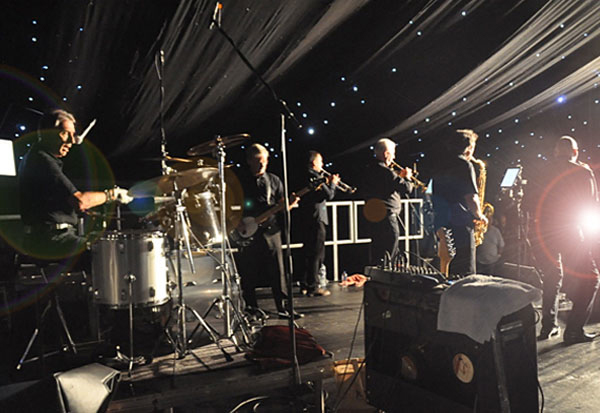October 13, 2023